Thermodynamic Potential MCQ Level – 2

# Thermodynamic Potential MCQ Level – 2

Test Description

## 10 Questions MCQ Test Topic wise Tests for IIT JAM Physics | Thermodynamic Potential MCQ Level – 2

Thermodynamic Potential MCQ Level – 2 for IIT JAM 2023 is part of Topic wise Tests for IIT JAM Physics preparation. The Thermodynamic Potential MCQ Level – 2 questions and answers have been prepared according to the IIT JAM exam syllabus.The Thermodynamic Potential MCQ Level – 2 MCQs are made for IIT JAM 2023 Exam. Find important definitions, questions, notes, meanings, examples, exercises, MCQs and online tests for Thermodynamic Potential MCQ Level – 2 below.
Solutions of Thermodynamic Potential MCQ Level – 2 questions in English are available as part of our Topic wise Tests for IIT JAM Physics for IIT JAM & Thermodynamic Potential MCQ Level – 2 solutions in Hindi for Topic wise Tests for IIT JAM Physics course. Download more important topics, notes, lectures and mock test series for IIT JAM Exam by signing up for free. Attempt Thermodynamic Potential MCQ Level – 2 | 10 questions in 45 minutes | Mock test for IIT JAM preparation | Free important questions MCQ to study Topic wise Tests for IIT JAM Physics for IIT JAM Exam | Download free PDF with solutions
 1 Crore+ students have signed up on EduRev. Have you?
Thermodynamic Potential MCQ Level – 2 - Question 1

### In a pressure cooker, the vapour pressure is greater than 1 atm. Under such condition, the boiling temperature of water will be Select one:

Detailed Solution for Thermodynamic Potential MCQ Level – 2 - Question 1

From Claussius-Clapeyron eauation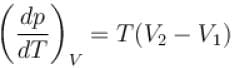on boiling V2 > V1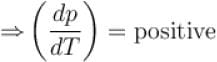Hence if we raise pressure above 1 atm the boiling point will also increase and will be higher than 373 K.
The correct answer is: Higher than 373 K

Thermodynamic Potential MCQ Level – 2 - Question 2

### On a p-T graph, the curve which connects points at which vapour (v) and solid (s) exists in equilibrium called Select one:

Detailed Solution for Thermodynamic Potential MCQ Level – 2 - Question 2

Sublimation curve connects points at which vapour and solid exists in equilibrium.
The correct answer is: Sublimation curve

Thermodynamic Potential MCQ Level – 2 - Question 3

### In one of the Maxwell’s relations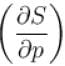equals Select one:

Detailed Solution for Thermodynamic Potential MCQ Level – 2 - Question 3

From Maxwell’s equations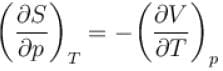The correct answer is: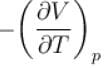Thermodynamic Potential MCQ Level – 2 - Question 4

Entropy of a system having average energy E and partition function Z is defined as
Select one:

Detailed Solution for Thermodynamic Potential MCQ Level – 2 - Question 4

F = –kT ln Z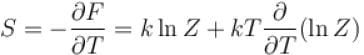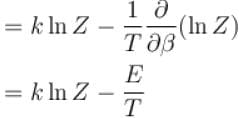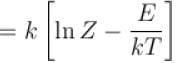= k[ln Z – βE]
The correct answer is: S = k[ln(Z) – βE]

Thermodynamic Potential MCQ Level – 2 - Question 5

Helmholtz free energy, F = U – TS. Which relation holds true? (where U = Internal energy; S = Entropy)
Select one:

Detailed Solution for Thermodynamic Potential MCQ Level – 2 - Question 5

F = U – TS
⇒ dF = dU – TdS – SdT
dF = –pdV – SdT ...(1)
take V constant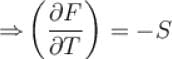take T constant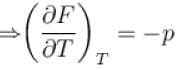The correct answer is: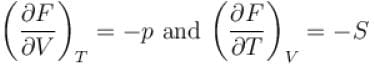Thermodynamic Potential MCQ Level – 2 - Question 6

Which of the following is true with regards to critical point?
Select one:

Detailed Solution for Thermodynamic Potential MCQ Level – 2 - Question 6

Critical point is that point on vapourization curve at which liquid and vapour phase have same density and above this temperature, a gas cannot be liquified by increasing the pressure.
The correct answer is: Above critical point, a gas cannot be liquified

Thermodynamic Potential MCQ Level – 2 - Question 7

Helmholtz free energy function and internal energy U are related as
Select one:

Detailed Solution for Thermodynamic Potential MCQ Level – 2 - Question 7

F = U – TS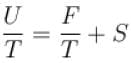differentiating w.r.t. ‘T’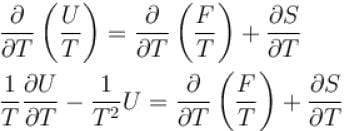keeping V constant, dW = 0
⇒dU = dQ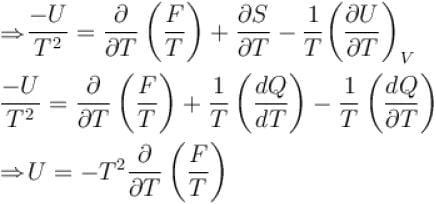The correct answer is: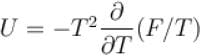Thermodynamic Potential MCQ Level – 2 - Question 8

Which of the following relations between internal energy U and the canonical partition function Z is true?
Select one:

Detailed Solution for Thermodynamic Potential MCQ Level – 2 - Question 8

Internal energy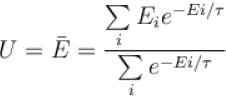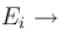Energy of the system in the ith state the partition function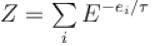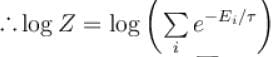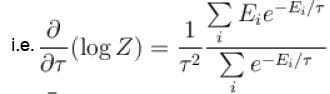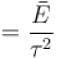Thus,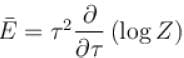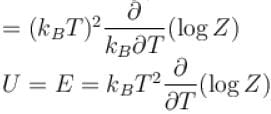The correct answer is: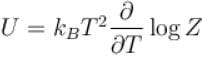Thermodynamic Potential MCQ Level – 2 - Question 9

Consider two level question system with energies ε1 = 0, ε2 = ε, The Helmholtz free energy of the system is given by
Select one:

Detailed Solution for Thermodynamic Potential MCQ Level – 2 - Question 9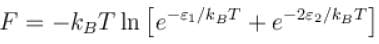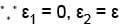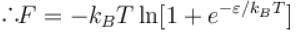The correct answer is: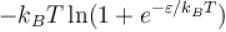Thermodynamic Potential MCQ Level – 2 - Question 10

The boiling point of water will decrease as we go to a higher altitude because
Select one:

Detailed Solution for Thermodynamic Potential MCQ Level – 2 - Question 10

As we go to a higher altitude, the pressure decreases and from ClaussiusClapeyron equation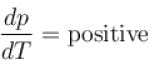Since dp is negative dT has to be negative hence boiling point decreases.
The correct answer is: Pressure decreases

## Topic wise Tests for IIT JAM Physics

217 tests
 Use Code STAYHOME200 and get INR 200 additional OFF Use Coupon Code
Information about Thermodynamic Potential MCQ Level – 2 Page
In this test you can find the Exam questions for Thermodynamic Potential MCQ Level – 2 solved & explained in the simplest way possible. Besides giving Questions and answers for Thermodynamic Potential MCQ Level – 2, EduRev gives you an ample number of Online tests for practice

217 tests